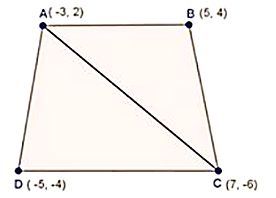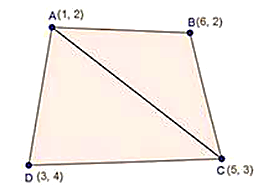# RD Sharma Class 10 Ex 14.5 Solutions Chapter 14 Coordinate Geometry

In this chapter, we provide RD Sharma Class 10 Ex 14.5 Solutions Chapter 14 Coordinate Geometry for English medium students, Which will very helpful for every student in their exams. Students can download the latest RD Sharma Class 10 Ex 14.5 Solutions Chapter 14 Coordinate Geometry pdf, Now you will get step by step solution to each question.

# Chapter 14: Coordinate Geometry Exercise – 14.5

### Question: 1

Find the area of a triangles whose vertices are

(i) (6, 3), (-3, 5) and (4, – 2)

(ii) [(at12, at1),( at22, 2at2)( at32, 2at3)]

(iii) (a, c + a), (a, c) and (-a, c – a)

### Solution:

(i) Area of a triangle is given by

1/2[x1(y– y3) + x2(y– y1) + x3(y+ y2)]

Here, x= 6,y= 3, x2 = -3, y= 5, x= 4,y3 = -2]

Let A(6, 3), B(-3, 5) and C(4,-2) be the given points

Area of ∆ABC = 1/2 [6(5+2)+(-3)(- 2 -3)+ 4(3 – 5)]

=1/2 [6 × 7- 3 × ( – 5) + 4( – 2)]

= 1/2[42 +15 – 8]

= 49/2 sq. units

(ii) Let A = (x1, y­1) = (at12, 2at1)

B = (x2,y2) = (at22, 2at2)

= (x3, y3) = (at32, 2at2) be the given points.

The area of ∆ABC

(iii) Let A = (x1,y1) = (a, c + a)

B = (x2, y2) = (a, c)

C = (x3, y3) = (- a, c – a) be the given points

The area of ∆ABC

= 1/2[a ( – {c – a}) + a(c – a – (c + a)) +( – a)(c + a – a)]

= 1/2 [a(c – c + a) + a(c – a – c – a) – a(c + a – c)]

= 1/2[a × a + ax( – 2a) – a × a]

= 1/2[a– 2a– a2]

= 1/2×(-2a)2

= – a2

### Question: 2

Find the area of the quadrilaterals, the coordinates of whose vertices are

(i) (-3, 2), (5, 4), (7, -6) and (-5,- 4)

(ii) (1, 2), (6, 2), (5, 3) and (3, 4)

(iii) (-4, -2), (-3, -5), (3, -2), (2, 3)

### Solution:

(i) Let A(-3, 2), B(5, 4), C(7,- 6) and D ( -5, – 4) be the given points.Area of ∆ABC

= 1/2[-3(4 + 6) + 5(- 6 – 2) + 7(2 – 4)]

= 1/2[-3×1 + 5×(-8) + 7(-2)]

= 1/2[- 30 – 40 -14]

=  – 42

But area cannot be negative

∴  Area of ∆ADC = 42 square units

= 1/2[-3( – 6 + 4) + 7(- 4 – 2) + (- 5)(2 + 6)]

= 1/2[- 3( – 2) + 7(- 6) – 5 × 8]

= 1/2[6 – 42 – 40]

= 1/2 × – 76

= – 38

But area cannot be negative

∴ Area of ∆ADC = 38 square units

= Ar. of ABC+ Ar of ADC

= (42 + 38)

= 80 square. Units

(ii)  Let A(1, 2) , B (6, 2) , C (5, 3) and (3, 4) be the given pointsArea of ∆ABC

= 1/2[1(2 – 3) + 6(3 – 2) + 5(2 – 2)]

= 1/2[ -1 + 6 × (1) + 0]

= 1/2[ – 1 + 6]

= 5/2

= 1/2[1(3 – 4) + 5(4 – 2) + 3(2 – 3)]

= 1/2[-1 × 5 × 2 + 3(-1)]

= 1/2[-1 + 10 – 3]

= 1/2

= 3

= Area of ABC + Area of ADC

(iii) Let A (- 4, 2), B( – 3, – 5), C (3,- 2) and D(2, 3) be the given pointsArea of ∆ABC = 1/2|(- 4)(- 5 + 2) – 3(-2 + 2) + 3(- 2 + 5)|

= 1/2|(-4)(-3) – 3(0) + 3(3)|

=  21/2

Area of ∆ACD = 1/2|( – 4)(3 + 2) + 2( – 2 + 2) + 3( – 2 – 3)|

= 1/2|- 4(5) + 2(0) + 3(- 5)|= (- 35)/2

But area can’t neative, hence area of ∆ADC = 35/2